# Texas Go Math Grade 6 Lesson 12.1 Answer Key Writing Equations to Represent Situations

Refer to our Texas Go Math Grade 6 Answer Key Pdf to score good marks in the exams. Test yourself by practicing the problems from Texas Go Math Grade 6 Lesson 12.1 Answer Key Writing Equations to Represent Situations.

## Texas Go Math Grade 6 Lesson 12.1 Answer Key Writing Equations to Represent Situations

Determine whether the given value is a solution of the equation.

Question 1.
11 = n + 6; n = 5
Given equation:
11 = n + 6
Substitute the value of n in the given equation:
11 = 5 + 6
Simplify:
11 = 11
Since the left and right hand side of the equation are equal, this implies that the given value of the variable is the solution of the given equation.
n = 5 is the solution of the given equation.

Question 2.
y – 6 = 24; y = 18
Given equation:
y – 6 = 24
Substitute the value of y in the given equation:
18 – 6 = 24
Simplify:
12 = 24
But:
12 ≠ 24
Since the left and right hand side of the equation are not equal this implies that the given value of the variable is not the solution of the given equation.
y = 18 is not the solution of the given equation.

Question 3.
$$\frac{36}{x}$$ = 9; x = 4
Substitute the value to evaluate the equation.
$$\frac{36}{4}$$ = 9 substitute for the value of x
9 = 9 the value of x is a solution to the equation
The given value of 4 is a solution to the equation.

Write an equation to represent each situation.

Question 4.
Marilyn has a fish tank that contains 38 fish. There are 9 goldfish and f other fish.
Solution to this example is given below38 = 9 + f

Question 5.
Juanita has 102 beads to make n necklaces. Each necklace will have 17 beads.
There are a total of 102 beads to make n necklaces and each must contain 17 beads, so the equation representing this situation becomes: $$\frac{102}{n}$$ = 17.

Question 6.
Craig is c years old. His 12-year-old sister Becky is 3 years younger than Craig.
Craig is 3 years older than his 12 year old sister, so his age is c = 12 + 3.

Question 7.
Sonia rented ice skates for h hours. The rental fee was $2 per hour and she paid a total of$8.
Solution to this example is given below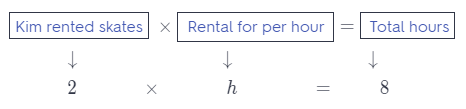2 × h = 8

Example

Sarah used a gift card to buy $47 worth of groceries. Now she has$18 left on her gift card. Write an equation to determine whether Sarah had $65 or$59 on the gift card before buying groceries.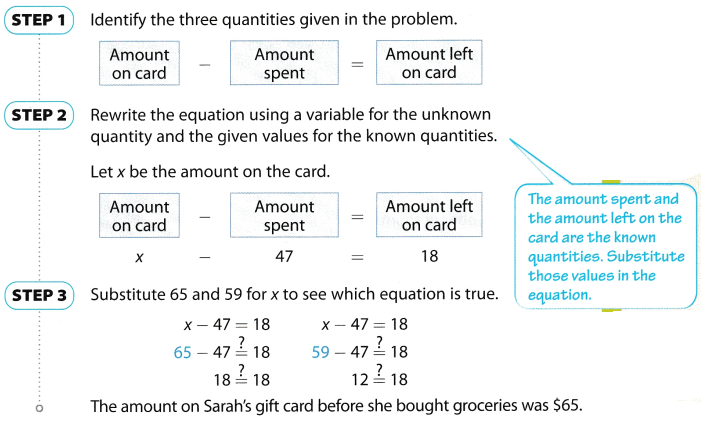Reflect

Question 8.
What expressions are represented in the equation x – 47 = 18? How does the relationship represented in the equation help you determine if the equation is true?
The expression represented in the equation is 47 less than the amount of the card. The relationship represented in the equation became true when the value of the card was substituted. Based from the substitution done, 65 is a solution to the equation and not 59.

Question 9.
On Saturday morning, Owen earned $24 raking leaves. By the end of the afternoon he had earned a total of$62. Write an equation to determine whether Owen earned $38 or$31 on Saturday afternoon.
Let the unknown amount earned be x, then the equation of total earning becomes:
x + 24 = 62
Solve for x:
x = 62 – 24 = 38
$38 were earned on Saturday afternoon. Texas Go Math Grade 6 Lesson 12.1 Guided Practice Answer Key Determine whether the given value is a solution of the equation. (Example 1) Question 1. 23 = x – 9; x = 14 __________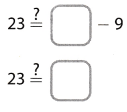Answer: Solution to this example is given below 23 = x – 9; x = 14 23 = 14 – 9 Substitute 14 for x 23 = 5 Subtract. 14 is a not of solution of the equation 23 = x – 9 x ≠ 14 Question 2. $$\frac{n}{13}$$ = 4; n = 52 ___________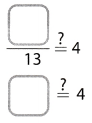Answer: Solution to this example is given below $$\frac{n}{13}$$ = 4; n = 52 $$\frac{52}{13}$$ = 4 Substitute 52 for n 4 = 4 Divide 52 is a solution of $$\frac{n}{13}$$ = 4 n = 52 Question 3. 14 + x = 46; x = 32 ___________ Answer: Solution to this example is given below 14 + x = 46; x = 32 14 + 32 = 46 Substitute 32 for x 46 = 46 Add 32 is a solution of 14 + x = 46 x = 32 Question 4. 17y = 85; y = 5 ___________ Answer: Solution to this example is given below 17y = 85; y = 5 17(5) = 85 Substitute 5 for y 85 = 85 Multiply. 5 is a solution of 17y = 85 y = 5 Question 5. 25 = $$\frac{k}{5}$$; k = 5 ___________ Answer: Given equation: 25 = $$\frac{k}{5}$$ Substitute the given value of k in the given equation: 25 = $$\frac{5}{5}$$ Simplify: 25 = 1 Compare: 25 ≠ 1 Since the left and right hand side of the equation are not equal, this implies that the given value of the variable is not a solution of the given equation. k = 5 is not a solution of the given equation. Question 6. 2.5n = 45; n = 18 ____________ Answer: Given equation: 2.5n = 45 Substitute the given value of n in the given equation: 2.5(18) = 45 Simplify: 45 = 45 Since the left and right hand side of the equation are equal, this implies that the given value of the variable is a solution of the given equation. n = 18 is a solution of the given equation. Question 7. 21 = m + 9; m = 11 ___________ Answer: Solution to this example is given below 21 = m + 9; m = 11 21 = 11 + 9 Substitute 11 for m 21 = 20 Add 11 is a not of solution of the equation 21 = m + 9 m ≠ 11 Question 8. 21 – h = 15; h = 6 ____________ Answer: Solution to this example is given below 21 – h = 15; h = 6 21 – 6 = 15 Substitute 6 for h 15 = 15 Subtract. 6 is a solution of 21 – h = 15 h = 6 Question 9. d – 4 = 19; d = 15 _____________ Answer: Given equation: d – 4 = 19 Substitute the given value of d in the given equation: 15 – 4 = 19 Simplify: 11 = 19 Compare: 11 ≠ 19 Since the left and right hand side of the equation are not equal, this implies that the given value of the variable is not a solution of the given equation. d = 15 is not a solution of the given equation. Question 10. 5 + x = 47; x = 52 ____________ Answer: Solution to this example is given below 5 + x = 47; x = 52 5 + 52 = 47 Substitute 52 for x 57 = 47 Add 52 is a not of solution of the equation 5 + x = 47 x ≠ 52 Question 11. w- 9 = 0; w = 9 ____________ Answer: Solution to this example is given below w – 9 = 0; w = 9 9 – 9 = 0 Substitute 9 for w 0 = 0 Subtract. 9 is a solution of w – 9 = 0 w = 9 Question 12. 5q = 31; q = 13 _____________ Answer: Given equation: 5q = 31 Substitute the given value of q in the given equation: 5(13) = 31 Simplify: 65 = 31 Compare: 65 ≠ 31 Since the left and right hand side of the equation are not equal, this implies that the given value of the variable is not a solution of the given equation. q = 13 is not a solution of the given equation. Question 13. Each floor of a hotel has r rooms. On 8 floors, there are a total of 256 rooms. Write an equation to represent this situation. (Example 2)Answer: Solution to this example is given below8 × r = 256 Question 14. In the school band, there are 5 trumpet players and f flute players. There are twice as many flute players as there are trumpet players. Write an equation to represent this situation. (Example 3) Answer: There are twice as many flute players as there are trumpet players, so f = 2 × 5 Question 15. Pedro bought 8 tickets to a basketball game. He paid a total of$208. Write an equation to determine whether each ticket cost $26 or$28. (Example 3)
Let the cost of 1 tickets = be x then the equation of total cost is:
8 × x = 208
Solve for x:
x = $$\frac{208}{8}$$
x = 26
Each ticket costs $26. Question 16. The high temperature was 92°F. This was 24°F higher than the overnight low temperature. Write an equation to determine whether the low temperature was 62°F or 68°F. (Example 3) Answer: Let the low temperature be x then the equation for high temperature is: x + 24 = 92 Solve for x: x = 92 – 24 Evaluate: x = 68 The low temperature was 68°F. Essential Question Check-In Question 17. Tell how you can determine whether a number is a solution of an equation. Answer: A number is checked if it is a solution of an equation or not by substituting its value in the equation and simplifying it. If the left and right hand side of the equation are equal then the number is a solution of the equation otherwise not. Texas Go Math Grade 6 Lesson 12.1 Independent Practice Answer Key Question 18. Andy is one-fourth as old as his grandfather, who is 76 years old. Write an equation to determine whether Andy is 19 or 22 years old. Answer: Let Andy’s age be x, then the equation becomes: x = 76 × $$\frac{1}{2}$$ Solve for x: x = 19 Andy is 19 years old. Question 19. A sleeping bag weighs 8 pounds. Your backpack and sleeping bag together weigh 31 pounds. Write an equation to determine whether the backpack without the sleeping bag weighs 25 or 23 pounds. Answer: Let the weight of the backpack be x, then the equation becomes: x + 8 = 31 Solve for x: x =31 – 8 Evaluate: x = 23 The sleeping bag weighs 23 pounds. Question 20. Halfway through a bus route, 23 students have been dropped off and 48 students remain on the bus. Write an equation to determine whether there are 61 or 71 students on the bus at the beginning of the route. Answer: Let the total number of students on the bus be x, then the equation becomes: x = 23 + 48 Solve for x: x = 71 There are 71 students on the bus at the beginning of the route. Question 21. The table shows the distance between Greenville and nearby towns. The distance between Artaville and Greenville is 13 miles less than the distance between Greenville and Jonesborough.a. Write two equations that state the relationship of the distances between Greenville, Artaville, and Jonesborough. Answer: Equations x = 29 – 13 and 29 = 13 + x x is the distance between Greenville and Artaville 29 is the distance between Greenville and Jonesborough 13 Less than the distance between Greenville and Jonesborough b. Describe what your variable represents. Answer: The variable x represents the distance between Greenville and Artaville. Question 22. Write an equation that involves multiplication, contains a variable, and has a solution of 5. Can you write another equation that has the same solution and includes the same variable and numbers but uses division? If not, explain. If possible, write the equation. Answer: Possible equations are: 15x = 75 and x = $$\frac{75}{15}$$ which both have a solution of 5 Question 23. How are expressions and equations different? Explain using a numerical example. Answer: An expression represents a single value can be evaluated ¡f there ¡s an indicated value of the variable while an equation shows an equality of two expressions which may be true for some values. Expression: 29 – 3x or 7x + 8y Equation: 29 – 3x = 35 or 7x + 8 = 1 Expression indicates a single value while equation shows equality between expressions. Question 24. Explain the Error The problem states that Ursula earns$9 per hour. To write an expression that tells how much money Ursula earns for h hours, Joshua wrote $$\frac{9}{h}$$. Sarah wrote 9h. Whose expression is correct and why?
Here the rate is given and the number of hours are given, so the total earning is a product of these 2, that is $9 × h =$9h. so Sarah is correct.

Question 25.
Communicate Mathematical Ideas A dog weighs 44 pounds and the veterinarian thinks it needs to lose 7 pounds. Mikala wrote the equation x + 7 = 44 to represent the situation. Kirk wrote the equation 44 – x = 7. Which equation is correct? Can you write another equation that represents the situation?
Kirk’s equation 44 – x = 7 is correct because the final weight x of the dog must be less than 44 pounds by 7 pounds.
This situation can also be represented by the equation: x = 44 – 7.
Kirk is correct.

Question 26.
Multiple Representations The table shows ages of Cindy and her dad.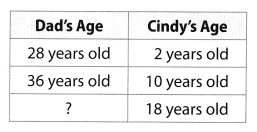a. Write an equation that relates Cindy’s age to her dad’s age when Cindy is 18.
Equation
y = x + 26
y is the age of the dad of Cindy
x is the age of Cindy
26 is the difference of their ages

b. Determine if 42 is a solution to the equation. Show your work.
y = x + 26 substitute for the value of x and y
42 = 18 + 26 add the numbers
42 ≠ 44
42 is not a solution to the equation

Based from the previous solution, it shows that 42 is not a solution to the equation It indicates that the dad of Cindy is not 42 years old when Cindy is 18 years old.
The age of the dad of Cindy is not 42 when she is 18 years old. Her dad is 44 years old.

H.O.T. Focus on Higher Order Thinking

Question 27.
Critical Thinking In the school band, there are 4 trumpet players and f flute players. The total number of trumpet and flute players is 12. Are there twice as many flute players as trumpet players? Explain.
Problem Solving Ronald paid $162 for 6 tickets to a basketball game. During the game he noticed that his friend paid$ 130 for 5 tickets. The price of each ticket was $26. Was Ronald overcharged? Justify your answer. Answer: If each tickets costs$26. then the cost of 6 tickets should he 6 × $26 =$156 < $162. Therefore, it can be said that Ronald was overcharged by$162 – $156 =$6.